Time Value of Money - Six Functions of a Dollar
Using Assessors Handbook Section 505 (Capitalization Formulas and Tables)
Appraisal Training: Self-Paced Online Learning Session

# Lesson 1: Overview

This lesson serves as an introduction to the topic and discusses the following:

• The concept of the time value of money,
• Timelines for cash flows,
• Simple versus compound interest, and
• Use of Assessors´ Handbook Section 505, Capitalization Formulas and Tables.

## Objectives and Format of Learning Session

The objectives of this learning session are to:

• Provide an understanding of the time value of money.
• Explain the compound interest functions presented in Assessors' Handbook Section 505, Capitalization Formulas and Tables, and their relationship to appraisal.
• Demonstrate how to use the factor tables and each compound interest function in Assessors' Handbook Section 505, to estimate the market value of property for property tax purposes.

In this learning session, instruction is provided through structured reading and illustrated examples. To assist in the attainment of the learning objectives, examples are incorporated within the lessons to illustrate the concept being discussed. Problems available at the end of lessons are to be worked by participants to ensure comprehension of concepts and calculations discussed in the lesson. A final examination is also available at the conclusion of this session, in order that certified property tax appraisers employed by a California County Assessors Office or the Board of Equalization may demonstrate their overall comprehension of the subject matter, attest to their participation in the learning session and receive training credit for completion of the session.

## The Concept of the Time Value of Money

It´s intuitive to most people that a dollar today is preferable to a dollar to be received in the future. (Think about \$1,000,000 today compared to \$1,000,000 to be received 5 years from today. Which would you rather have?)

A dollar today can be invested to accumulate to more than a dollar in the future, which also makes a future dollar worth less than a dollar today. Hence, money has a time value. More generally, the time value of money is the relationship between the value of a payment at one point in time and its value at another point in time as determined by the mathematics of compound interest.

Because of the time value of money, payments made at different points in time cannot be directly compared. The compound interest functions—the mathematics of the time value of money – allow us to bring the payments to the same point in time for comparison purposes.

Time value of money calculations have wide application in finance, real estate, and personal financial decisions. An understanding of them is essential in the field of valuation. There are several situations in real estate where dollars at different points in time are compared:

• An investor buys a property today for a certain amount of money in order to receive expected income from the property in the future (the purchase price today is compared to the expected future income stream).
• A lender makes a loan today in exchange for a promise by the borrower to pay scheduled amounts in the future (the loan amount is compared to the promised payments).
• A property owner wants to prepare for a future estimated expense by setting aside a given amount of money each month or year (the future expense is compared to the money set aside each year).
• An investor expects that a property will be worth a certain amount in the future and wants to estimate the equivalent amount today (the expected future value is compared to a present value).

The process by which payments (or cash flows) are moved forward in time is called compounding. The process by which payments (or cash flows) are moved backward in time is called discounting. All time value of money calculations involve either compounding or discounting — that is, moving amounts either forward or backward in time.

## Timelines for Cash Flows

A series of cash flows can be graphically represented using a cash flow timeline. A timeline depicts the timing and amount of the cash flows.

For example, the following timeline depicts cash inflows of \$100 to be received at the end of each of the next 5 years: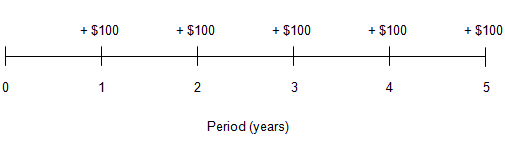Cash flows in a timeline are often labeled positive or negative. By convention, positive cash flows correspond to cash inflows; negative cash flows correspond to cash outflows.

Whether a cash flow is an inflow or an outflow depends on perspective (i.e., as a borrower or lender). The borrower´s inflow is the lender´s outflow, and vice versa.

In using timelines, and in solving time value of money problems, one should adopt the perspective of either borrower or lender and stay with that perspective throughout the problem.

Consider a simple time value of money problem. In making a purchase you are given two payment alternatives:

• Pay \$400 immediately.
• Pay five installments of \$100 each at the end of each of the next five years.

As depicted on a cash flow timeline: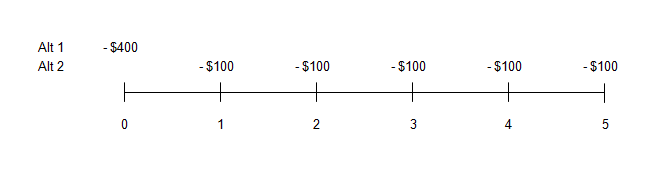In deciding which alternative is better, we can´t simply add up the five payments of \$100 and compare this sum (\$500) with alternative 1 (\$400 today). To do so would ignore the time value of money because the two alternatives involve payments at different times. Instead, we must determine the value today (at time 0) of the five future payments of \$100 of alternative 2 and compare this to \$400, which is the value today of alternative 1. As we shall see, determining the value today (the present value) of the five payments under alternative 2 involves calculating the present value of those payments at a given rate of interest.

Time value of money calculations allow us to solve problems such as the one above and many others.

## Simple versus Compound Interest

When money is borrowed, the amount borrowed is called the principal. The consideration paid for the use of money is called interest.

• The rate of interest can be thought of as a price per period for the use of money.
• From the perspective of the lender, interest is earned; from the perspective of the borrower, interest is paid.

Simple Interest

Simple interest refers to the situation in which interest is calculated on the original principal amount only.

With simple interest, the base on which interest is calculated does not change, and the amount of interest earned each period also does not change.

Compound Interest

Compound interest refers to the situation in which interest is calculated on the original principal and the accumulated interest.

With compound interest, interest is calculated on a base that increases each period, and the amount of interest earned also increases with each period.

Application of Simple Interest

Suppose someone invests \$100 for 50 years and receives 5% per year in simple interest. To calculate simple interest, multiply the beginning balance by the rate 0.05 × \$100 = \$5. The growth in the investment is depicted in the table below: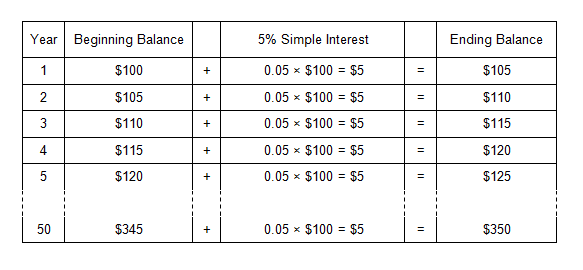With simple interest, each year´s interest is based on the original principal amount only.

Application of Compound Interest

Assume the same investment of \$100 for 50 years, but at compound interest: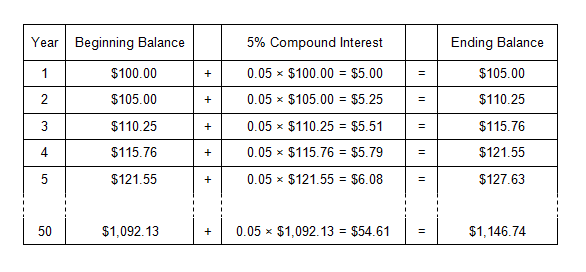With compound interest, interest is earned on both the original principal and accumulated interest. Interest is earned on interest.

In the preceding example, with simple interest, the accumulated amount after 50 years is only \$350. With compound interest, the accumulated amount is \$1,147. As the term increases, the difference between the final amount with compound interest versus simple interest becomes more and more dramatic.

"Miracle of Compound Interest"

In a well-known transaction, Dutch colonists bought Manhattan Island in 1624 for the equivalent of \$24.

• This seems like a steal, but if the seller had deposited the \$24 and earned an annual rate of 6%, the future compound amount would have been about \$141 billion in the year 2010.
• This is roughly equal to the total assessed value of all land and improvements in the City and County of San Francisco in the year 2010.
• Over the same time period (386 years), the future value of \$24 at simple interest of 6% would have been only \$580.

From a lender´s (or investor´s) perspective, compound interest is a good thing; the lender earns interest on interest from the borrower. Conversely, from a borrower´s perspective, compound interest is not so good. The borrower, in effect, pays interest on interest throughout the term of the loan.

Compound Interest Functions

Six compound interest functions are used to solve time value of money problems. Not surprisingly, all of the functions are based on compound, not simple, interest. Each compound interest function is defined by a formula, which is the basis for calculating the compound interest factors for that function. Each formula requires a periodic interest rate and the number of periods

Most time value of money problems involve the use of only one compound interest function (or factor), but some require the use of two or more.

Understanding the compound interest functions, and how the factors derived from them are used to solve time value of money problems, is the heart of this subject matter. Each compound interest formula, and the factors derived from it, involves three variables:

1. An interest rate,
2. A term (number of periods), and
3. A compounding interval (how frequently interest is compounded).

In essence, using a compound interest factor does one of two things:

1. Adds compound interest to a present value to arrive at a future value.
2. Subtracts compound interest from a future value to arrive at a present value.

Published tables of compound interest factors are used to solve time value of money problems. It´s easier to refer to a table of factors than to calculate the desired factor from one of the formulas each time you need it.

Time value of money problems can also be solved using a financial calculator or spreadsheet software. Essentially, the software calculates the necessary factor and processes the calculation.

We approach the subject by first showing how compound interest factors are derived from each of the formulas, then showing how the factors are used to solve various time value of money problems.

This approach provides a fundamental understanding of the material and a good basis for later using financial calculators and spreadsheet applications to solve time value of money problems.

The six compound interest functions are listed below; the following table briefly describes each function and gives an example of how it might be used.

• Future Worth of \$1 (FW\$1)
• Present Worth of \$1 (PW\$1)
• Future Worth of \$1 Per Period (FW\$1/P)
• Sinking Fund Factor (SFF)
• Present Worth of \$1 Per Period (PW\$1/P)
• Periodic Repayment (PR)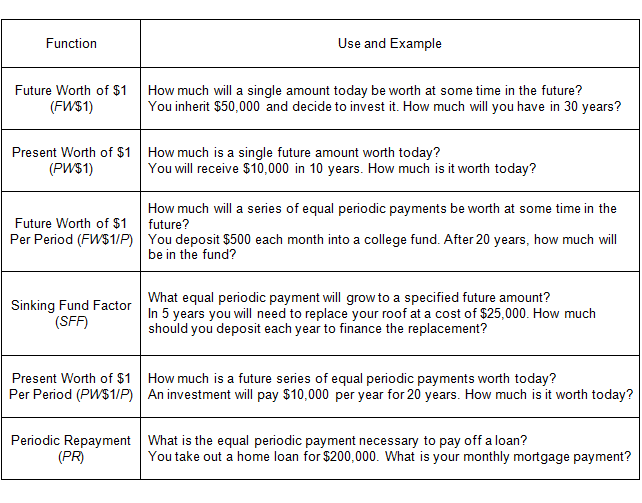Review the above table as an introduction to the compound interest functions. Note that the first two compound interest functions (FW\$1 and PW\$1) deal with a single amount, or payment, while the remaining four deal with a series of payments (an annuity). Although all of the compound interest functions have appraisal applications, two are of particular importance because of their central role in the income approach – the present worth of \$1 (PW\$1) and the present worth of \$1 per period (PW\$1/P).

## Using Assessors’ Handbook Section 505

Assessors´ Handbook Section 505 (AH 505), Capitalization Formulas and Tables, contains a set of compound interest factors for use by property tax appraisers.

• AH 505 begins with introductory material (pages 1-11) that describes the compound interest functions, shows their formulas, and demonstrates some sample problems. We will cover this material in the presentation.
• The remainder of AH 505 (pages 12-109) contains tables of compound interest factors over a range of interest rates for the six compound interest functions.
• AH 505 contains compound interest factors at interest rates from 1 to 25%, at 1/2 % intervals, for time periods up to 50 years. If a problem requires a term longer than 50 years, the tables can be extended using certain calculations.
• For each interest rate, there is a separate page for either annual or monthly compounding. The frequency of compounding (such as on a monthly basis versus annual) directly affects the present and future values in discounting – see separate lesson on Frequency of Compounding (Lesson 9).

Briefly look through AH 505 (Use browser's back button to return to lessons). To use the AH 505 tables, follow these steps:

1. Locate the page at the desired interest rate, selecting the page for either annual or monthly compounding (a common error is using the annual page when the monthly page is required, or vice versa).
2. Find the desired term (number of periods, months or years) by going down the far left side of the page.
3. Go across to the proper column to find the factor for the desired compound interest function.

(For solving the examples, we have recreated portions of the AH505 tables. We also provide a link to the actual pages in AH 505. If you go to an actual page, use the zoom feature for the best view.)

Example 1:
Find the FW\$1 factor at 10% for 10 years, given annual compounding.

Solution:
In AH 505:

• Search for the page at 10%, annual compounding, (step 1 above); the correct page is page 49.
• Go down 10 years and across to column 1 (FW\$1) (steps 2 and 3); the correct factor is 2.593742.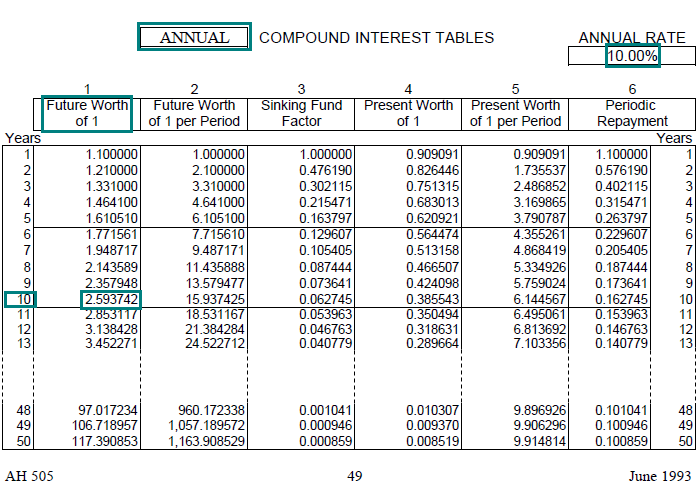Link to AH 505, page 49

Example 2:
Find the PW\$1 factor at 5% for 5 years, given monthly compounding.

Solution:
In AH 505:

• Search for the page at 5%, monthly compounding; the correct page is page 28.
• Go down 5 years and across to column 4 (PW\$1); the correct factor is 0.779205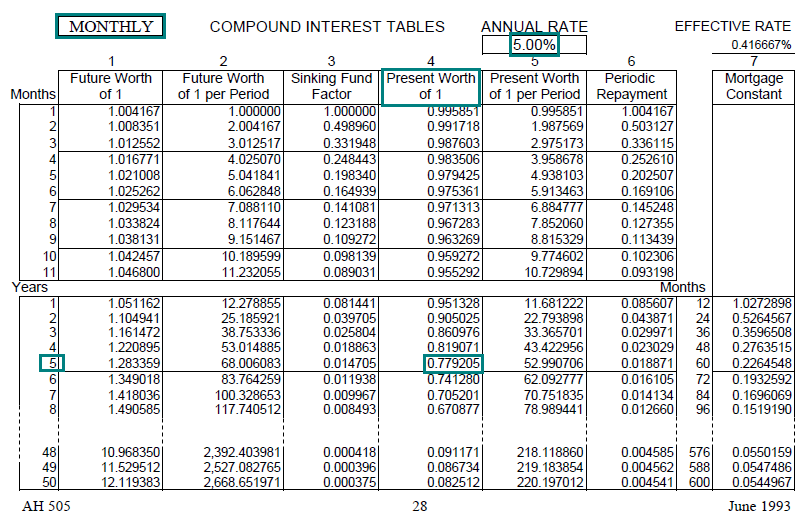Link to AH 505, page 28

Example 3:
Find the PW\$1/P factor at 12.5% for 8 years, given annual compounding.

Solution:
In AH 505:

• Search for the page at 12.5%, annual compounding; the correct page is page 59.
• Go down 8 years and across to column 5 (PW\$1/P); the correct factor is 4.882045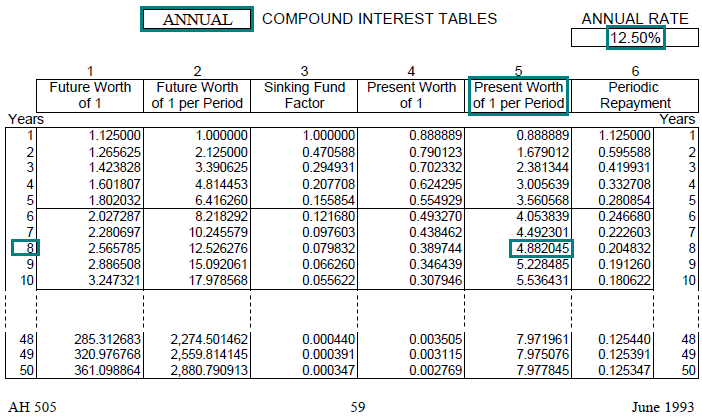Link to AH 505, page 59